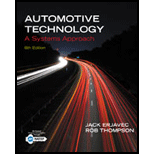Chapter 3, Problem 20RQAutomotive Technology: A Systems A...

6th Edition
Jack Erjavec + 1 other
ISBN: 9781133612315

Solutions

Chapter
SectionAutomotive Technology: A Systems A...

6th Edition
Jack Erjavec + 1 other
ISBN: 9781133612315
Textbook Problem

While discussing different types of energy: Technician A says that when energy is released to do work, it is called potential energy. Technician B says that stored energy is referred to as kinetic energy. Who is correct? Technician A only Technician B onlyBoth A and BNeither A nor B

To determine

The technician with correct approach on the different types of energy.

Explanation

Given information:

Technician A says that when energy is released to do work, it is called potential energy.

Technician B says that stored energy is referred to as kinetic energy.

Data, values, standard values, figures, and tables are mentioned in the given question.

Potential energy can be referred to as that energy which is stored in a body due to its height or position. For example, an object on the top of a building has potential energy due to the gravitational force acting on it. The more the mass of the object, more will be its potential energy. It is given by-

Potential Energy, PE=mgh

Where, ‘m’ is the mass of the object, ‘g’ is the gravitational force and ‘h’ is the height of the object.

Kinetic energy is referred to as the energy stored in the body due to its motion

Still sussing out bartleby?

Check out a sample textbook solution.

See a sample solution

The Solution to Your Study Problems

Bartleby provides explanations to thousands of textbook problems written by our experts, many with advanced degrees!

Get Started